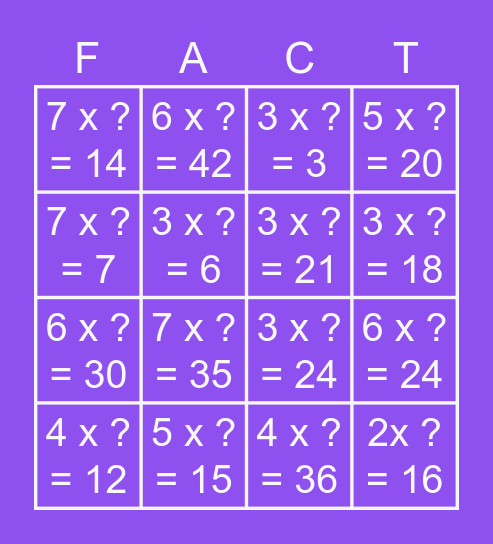# Missing FactorsThis bingo card has 16 words: 5 x ? = 15, 6 x ? = 24, 3 x ? = 3, 7 x ? = 14, 6 x ? = 30, 6 x ? = 42, 3 x ? = 24, 4 x ? = 36, 7 x ? = 7, 3 x ? = 6, 4 x ? = 12, 5 x ? = 20, 7 x ? = 35, 3 x ? = 18, 3 x ? = 21 and 2x ? = 16.

## Play Online